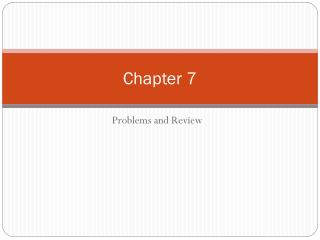DownloadDownload PresentationChapter 7

# Chapter 7

Download Presentation## Chapter 7

- - - - - - - - - - - - - - - - - - - - - - - - - - - E N D - - - - - - - - - - - - - - - - - - - - - - - - - - -
##### Presentation Transcript

1. Chapter 7 Problems and Review

2. How much are you willing to pay for these things and why? • Can of Coke • Pair of Louis Vuitton heels • Tickets to the Super bowl • A new shirt to wear to school

3. Graph the consumer surplus of a market that has an equilibrium price of \$5 and an equilibrium quantity of 100. • Graph the new surplus for when the price falls to \$3.

4. Question 1 • Mr. Zedan loves hotdogs. He can buy as many hotdogs he wishes at a price of \$0.70 per dog. On a particular day, he is willing to pay \$1.50 for the first dog, \$1.10 for the second dog, \$0.65 for the third dog, and \$0.50 for the fourth dog. Assume Mr. Wicker is rational in deciding how many dogs to buy. How many hotdogs will he buy? What is his consumer surplus?

5. He will buy 2 hotdogs. • His total consumer surplus is \$1.20 • 80 cents for the first one, 40 cents on the second • He would not buy the last dog because it cost more than he is willing to pay.

6. Question 2 • If the price of oranges decreases, what happens to consumer surplus in the market for orange juice? Why?

7. The increase in the supply of oranges would shift the supply of orange juice to the right, thus increasing consumer surplus.

8. Question 3 • Graph the consumer surplus of a market that has an equilibrium price of \$5 and an equilibrium quantity of 100.

9. Price A CS C B \$5 PS D 100 Q

10. Question 4 • What would be the be the designation of the area of consumer and producer surplus?

11. Price A \$10 • Consumer surplus: Triangle A,B,C • Producer Surplus: Triangle CBD CS C B \$5 PS D 100 Q

12. Question 5 • What is the value of consumer and producer surplus? What is total surplus?

13. They are each the same: \$250 • (5 * \$100)/2 = \$500/2 = \$250 • This is just the area of he triangle  • So \$250 + \$250 = \$500

14. Question 6 • What would happen to consumer surplus when the price is changed to \$7 and quantity to 70? Producer surplus? Total surplus? Is \$7 efficient? Equal?

15. Consumer surplus: \$105 \$10 Loss: \$60 Producer surplus: \$280 + \$105 = \$385 \$7 CS Not Equal Not Efficient \$5 PS \$3 D 70 100 Q

16. Question 7 • Answer the following questions based on the graph that represents J.R.'s demand for ribs per week of ribs at Judy's rib shack. • a. At the equilibrium price, how many ribs would J.R. be willing to purchase? • b. How much is J.R. willing to pay for 20 ribs? • c. What is the magnitude of J.R.'s consumer surplus at the equilibrium price? • d. At the equilibrium price, how many ribs would Judy be willing to sell? • e. How high must the price of ribs be for Judy to supply 20 ribs to the market? • f. At the equilibrium price, what is the magnitude of total surplus in the market? • g. If the price of ribs rose to \$10, what would happen to J.R.'s consumer surplus? • h. If the price of ribs fell to \$5, what would happen to Judy's producer surplus? • i. Explain why the graph that is shown verifies the fact that the market equilibrium (quantity) maximizes the sum of producer and consumer surplus.

17. a. 40 • b. \$10.00 • c. \$80.00. • d. 40 • e. \$5 • f. \$200 • g. It would fall from \$80 to only \$20. • h. It would fall from \$120 to only \$30. • i. At quantities less than the equilibrium quantity, the marginal value to buyers exceeds the marginal cost to sellers. Increasing the quantity in this region raises total surplus until equilibrium quantity is reached. At quantities greater than the equilibrium quantity, the marginal cost to sellers exceeds the marginal value to buyers and total surplus falls.

18. Question 8 • See question 9 in your textbook, page 156 